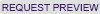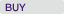# Case Detail

## Case Summary

### Practical Regression: Regression Basics

Case Number: 7-112-002, Year Published: 2012

HBS Number: KEL636### Key Concepts

Economics, Market Research, Statistical Methods

### Abstract

This is the second in a series of lecture notes which, if tied together into a textbook, might be entitled “Practical Regression.” The purpose of the notes is to supplement the theoretical content of most statistics texts with practical advice based on nearly three decades of experience of the author, combined with over one hundred years of experience of colleagues who have offered guidance. As the title “Practical Regression” suggests, these notes are a guide to performing regression in practice.
This technical note reviews the basic elements of regression analysis. Students who read this note will be able to explain the fundamental principals of regression, including (1) regression as an alegbraic equation; (2) dummy variables and nonlinear terms; (3) R-squared and goodness of fit; and (4) reporting the significance and economic importance of regression coefficients. Having completed this note, students will be prepared to study the special topics in regression that are covered in other notes in this series.

### Learning Objectives

Students will learn the following:
-How to use basic algebra to interpret a regression equation
-What a categorical (dummy) variable is and how to interpret results of models that include them
-Slope dummies
-How to interpret (and not overinterpret) the R-squared statistic
-What it means for a cofficient to be statistically significant at some level p
-How to describe the economic importance of a regression result